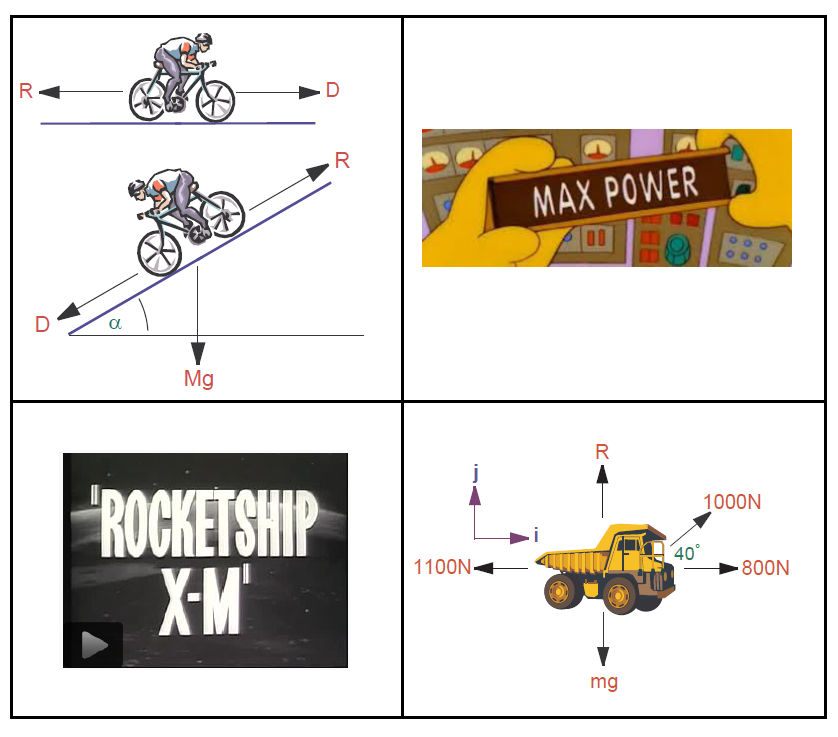### ACM 10080 Applied Mathematics: Mechanics and MethodsI will be teaching this module in 2022-2023.

Description: This course introduces students to the basic principles of Newtonian Mechanics. The module starts with a description of motion (kinematics) including notions of displacement, velocity, and acceleration. The module then moves on to an understanding of the causes of motion and changes in motion (dynamics) via an elementary introduction to Newton's laws. Key topics here include force and momentum. Concurrently with this discussion, there will be an introduction to elementary mathematical methods that can be used to frame all of the above in mathematical language, including the mathematical treatment of kinematics and dynamics via Calculus, vectors, Taylor series, and Ordinary Differential Equations.

Learning Outcomes: On completion of this module students should be able to:

1. Perform mathematical calculations using vectors, including vector addition, scalar multiplication, and dot products.
2. Use the Cartesian notation for vectors with proficiency.
3. Explain comprehensively the notions of vector, velocity, acceleration, momentum, kinetic energy, potential energy, moment of a force, center of mass, and moments of inertia.
4. Use Newton's laws to express and solve problems in mathematical terms.

### Module Documents

The pandemic has made us all rely more on proprietary technologies, owned by faceless unaccountable bureucratic corporations. This module is no exception and for that reason, the module will be delivered via the UCD Virtual Learning Environment for the year 2022-2023. However, the most up-to-date version of the lecture notes (2022) is made available here here as a resource.